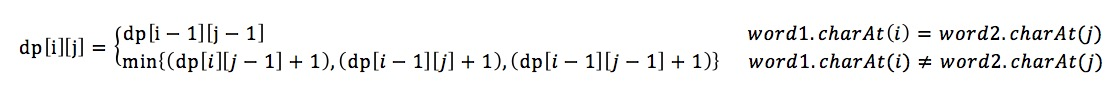Leetcode 72 Edit Distance

Leetcode 72 Edit DistanceCheck Edit Distance on GitHub

The problem description is as follow:

```Given two words word1 and word2, find the minimum number of steps required to convert word1 to word2. (each operation is counted as 1 step.)

You have the following 3 operations permitted on a word:

a) Insert a character
b) Delete a character
c) Replace a character```

This is a classical DP problem. I use a 2-D matrix `dp[][]` to save all the data.  `dp[i][j]` stands for the edit distance between two strings with length i and j, `word1[0,...,i-1]` and `word2[0,...,j-1]`.

Now we only have to figure how to get the recursive formula for `dp[i][j]`  from historical data we already got, which is equivalent to getting the relationship between `dp[i][j]`  and `dp[i-1][j-1]` . Let’s say we transform from `string word1` to `string word2`. The first string has length i and it’s last character is `word1.charAt(i) = x`; the second string has length and its last character is `word2.charAt(j) = y`.

```1. if x == y, then dp[i][j] == dp[i-1][j-1]
2. if x != y, and we insert y for word1, then dp[i][j] = dp[i][j-1] + 1
3. if x != y, and we delete x for word1, then dp[i][j] = dp[i-1][j] + 1
4. if x != y, and we replace x with y for word1, then dp[i][j] = dp[i-1][j-1] + 1
When x!=y, dp[i][j] is the min of the three situations.```And we have the initial condition as `dp[i] = i, dp[j] = j`

Here comes the code:

```public class Solution {
public int minDistance(String word1, String word2) {
int L1 = word1.length();
int L2 = word2.length();

// L1+1, L2+1, because finally return dp[L1][L2]
int[][] dp = new int[L1 + 1][L2 + 1];

for (int i = 0; i <= L1; i++) {
dp[i] = i;
}

for (int j = 0; j <= L2; j++) {
dp[j] = j;
}

//iterate though, and check last char
for (int i = 0; i < L1; i++) {
char c1 = word1.charAt(i);
for (int j = 0; j < L2; j++) {
char c2 = word2.charAt(j);

//if last two chars equal
if (c1 == c2) {
//update dp value for +1 length
dp[i + 1][j + 1] = dp[i][j];
} else {
int replace = dp[i][j] + 1;
int insert = dp[i][j + 1] + 1;
int delete = dp[i + 1][j] + 1;

int min = replace > insert ? insert : replace;
min = delete > min ? min : delete;
dp[i + 1][j + 1] = min;
}
}
}

return dp[L1][L2];
}
}```